# Track Stitching Example

## Introduction

Track Stitching considers a set of broken fragments of track (which we call tracklets), and aims to identify which fragments should be stitched (joined) together to form one track. This is done by considering the state of a tracked object and predicting its state at a future (or past) time. This example generates a set of tracklets, before applying track stitching. The figure below visualizes the aim of track stitching: taking a set of tracklets (left, black) and producing a set of tracks (right, blue/red).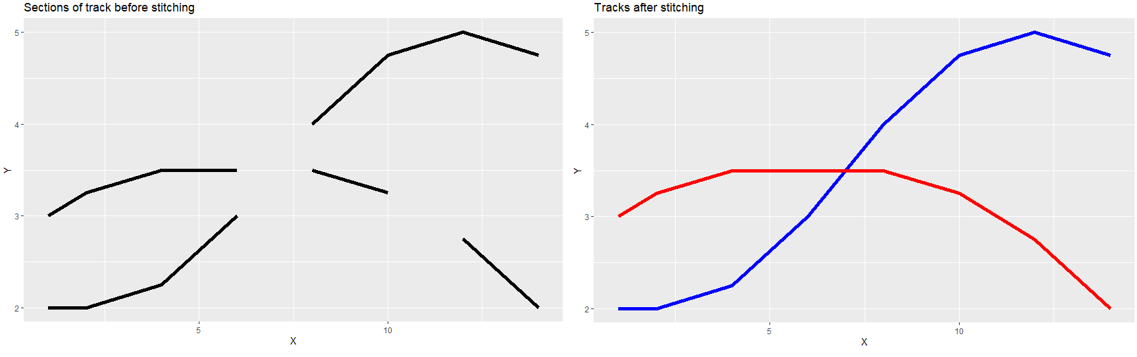### Track Stitching Method

Consider the following scenario: We have a bunch of sections of track that are all disconnected from each other. We aim to stitch the track sections together into full tracks. We can use the known states of tracklets at known times to predict where the tracked object would be at a different time. We can use this information to associate tracklets with each other. Methods of associating tracklets are explained below.

### Predicting forward

For a given track section, we consider the state at the end-point of the track, say state $$x$$ at the time that the observation was made, call this time $$k$$. We use the state of the object to predict the state at time $$k + \delta k$$. If the state at the start point of another track section falls within an acceptable range of this prediction, we may associate the tracks and stitch them together. This method is used in the function forward_predict.

### Predicting backward

Similarly to predicting forward, we can consider the state at the start point of a track section, call this time $$k$$, and predict what the state would have been at time $$k - \delta k$$. We can then associate and stitch tracks together as before. This method is used in the function backward_predict.

### Using both predictions

We can use both methods at the same time to calculate the probability that two track sections are part of the same track. The track stitcher in this example uses the KalmanPredictor to make predictions about which tracklets should be stitched into the same track.

### Import Modules

from datetime import datetime, timedelta
import numpy as np


## Scenario Generation

### Set Variables for Scenario Generation

The code below contains parameters used to generate input truth paths.

The number_of_targets is the total number of truth paths generated in the initial simulation.

The starting location of each truth path is defined in the region (-range_value, range_value) in all dimensions.

Each truth object is split into a number of segments chosen randomly from the range (1, max_segments).

You can define the minimum and maximum length that segments can be, by setting min_segment_length and max_segment_length, respectively.

Similarly, the length of disjoint sections can be bounded by min_disjoint_length and max_disjoint_length.

The start time of each truth path is bounded between $$t$$ = 0 and $$t$$ = max_track_start.

The simulation will run for any number of spacial dimensions, given by n_spacial_dimensions.

Finally, the transition model can be set by setting TM to either “CV” or “KTR” as indicated in the comments in the code below.

start_time = datetime.now().replace(second=0, microsecond=0)
np.random.seed(100)

number_of_targets = 10
range_value = 10000
max_segments = 10
max_segment_length = 125
min_segment_length = 60
max_disjoint_length = 250
min_disjoint_length = 125
max_track_start = 125
n_spacial_dimensions = 3
measurement_noise = 100

# Set transition model:
# ConstantVelocity = CV
# KnownTurnRate = KTR

TM = "CV"


### Transition and Measurement Models

The code below sets transition and measurement models. It also checks that sets of track data are empty before the scenario is generated.

from stonesoup.models.transition.linear import CombinedLinearGaussianTransitionModel, \
ConstantVelocity, KnownTurnRate
from stonesoup.models.measurement.linear import LinearGaussian

# Check all sets are empty
truths = set()
truthlets = set()
tracklets = set()
all_tracks = set()

# Set transition model
if TM == "CV":
transition_model = CombinedLinearGaussianTransitionModel([ConstantVelocity(1)] *
n_spacial_dimensions, seed=12)
elif TM == "KTR":
if n_spacial_dimensions != 2:
print("KnownTurnRate model only works for 2 dimensions. Changing from {} "
"dimensions to 2D.".format(n_spacial_dimensions))
n_spacial_dimensions = 2
else:
raise TypeError("Must assign 'CV' or 'KTR' to TM")

# Variable calculations for measurement model
measurement_cov_array = np.zeros((n_spacial_dimensions, n_spacial_dimensions), int)
np.fill_diagonal(measurement_cov_array, measurement_noise)

# Set measurement model
measurement_model = LinearGaussian(ndim_state=2 * n_spacial_dimensions,
mapping=list(range(0, 2 * n_spacial_dimensions, 2)),
noise_covar=measurement_cov_array)


### Generate ground truths and truthlets

Here we generate a set of ground truths. We then break the truths into alternating sections of truthlets (sections of ‘known’ state data) and disjoint sections (sections of no data). Note that no ‘truth’ data is used in track stitching - in this tutorial it is only used for generating tracklets and for evaluation of track stitching results.

from stonesoup.models.transition.linear import OrnsteinUhlenbeck
from stonesoup.predictor.kalman import KalmanPredictor
from stonesoup.updater.kalman import KalmanUpdater
from stonesoup.hypothesiser.distance import DistanceHypothesiser
from stonesoup.measures import Mahalanobis
from stonesoup.dataassociator.neighbour import GNNWith2DAssignment
from stonesoup.deleter.error import CovarianceBasedDeleter
from stonesoup.deleter.multi import CompositeDeleter
from stonesoup.deleter.time import UpdateTimeStepsDeleter
from stonesoup.initiator.simple import SimpleMeasurementInitiator
from stonesoup.types.groundtruth import GroundTruthPath, GroundTruthState
from stonesoup.types.state import GaussianState

# Parameters for tracker
predictor = KalmanPredictor(transition_model)
updater = KalmanUpdater(measurement_model)
hypothesiser = DistanceHypothesiser(predictor, updater, Mahalanobis(), missed_distance=30)
data_associator = GNNWith2DAssignment(hypothesiser)
deleter = CompositeDeleter([UpdateTimeStepsDeleter(50), CovarianceBasedDeleter(5000)])
initiator = SimpleMeasurementInitiator(
prior_state=GaussianState(np.zeros((2 * n_spacial_dimensions, 1), int),
np.diag([1, 0] * n_spacial_dimensions)),
measurement_model=measurement_model)
state_vector = [np.random.uniform(-range_value, range_value, 1),
np.random.uniform(-2, 2, 1)] * n_spacial_dimensions

# Calculate start and end points for truthlets given the starting conditions
for i in range(number_of_targets):
# Sets number of segments from range of random numbers
number_of_segments = int(np.random.choice(range(1, max_segments), 1))

# Set length of first truthlet segment
truthlet0_length = np.random.choice(range(max_track_start), 1)

# Set lengths of each of the truthlet segments
truthlet_lengths = np.random.choice(range(min_segment_length, max_segment_length),
number_of_segments)

# Set lengths of each disjoint section
disjoint_lengths = np.random.choice(range(min_disjoint_length, max_disjoint_length),
number_of_segments)

# Sum pairs of truthlets and disjoints, and set the start-point of the truth path
segment_pair_lengths = np.insert(truthlet_lengths + disjoint_lengths, 0, truthlet0_length,
axis=0)

# Cumulative sum of segments, giving the start point of each truth segment
truthlet_startpoints = np.cumsum(segment_pair_lengths)

# Sum truth segments length to start point, giving end point for each segment
truthlet_endpoints = truthlet_startpoints + np.append(truthlet_lengths, 0)

# Set start and end points for each segment
starts = truthlet_startpoints[:number_of_segments]
stops = truthlet_endpoints[:number_of_segments]
truth = GroundTruthPath([GroundTruthState(state_vector, timestamp=start_time)],
id=i)
for k in range(1, np.max(stops)):
truth.append(GroundTruthState(
transition_model.function(truth[k - 1], noise=True, time_interval=timedelta(seconds=1)),
timestamp=truth[k - 1].timestamp + timedelta(seconds=1)))
for j in range(number_of_segments):
truthlet = GroundTruthPath(truth[starts[j]:stops[j]], id=str("G::" + str(truth.id) +
"::S::" + str(j) + "::"))

print(number_of_targets, " targets required.")
print(len(truths), " truths have been generated.")
print(len(truthlets), " truthlets have been generated.")

/home/docs/checkouts/readthedocs.org/user_builds/stonesoup/checkouts/latest/docs/examples/Track_Stitcher_Example.py:186: DeprecationWarning:

Conversion of an array with ndim > 0 to a scalar is deprecated, and will error in future. Ensure you extract a single element from your array before performing this operation. (Deprecated NumPy 1.25.)

10  targets required.
10  truths have been generated.
56  truthlets have been generated.


### Generate a tracklet from each truthlet

We introduce measurement noise (as set in variables section) and generate a set of tracklets from the set of truthlets.

from stonesoup.tracker.simple import MultiTargetTracker
from stonesoup.types.detection import TrueDetection

# Generate tracklets from truthlets calculated above
for n, truthlet in enumerate(truthlets):
measurementlet = []
for state in truthlet:
m = measurement_model.function(state, noise=True)
m0 = TrueDetection(m,
timestamp=state.timestamp,
measurement_model=measurement_model,
groundtruth_path=truthlet)
measurementlet.append((state.timestamp, {m0}))
tracklet = MultiTargetTracker(initiator, deleter, measurementlet, data_associator, updater)
for _, t in tracklet:
all_tracks |= t

print(len(all_tracks), " tracklets have been produced.")

56  tracklets have been produced.


### Plot the set of tracklets

The following plots present the tracks which have been generated, as well as, for reference, the ground truths used to generate them. A 2D graph is plotted for each 2D plane in the N-D space.

from stonesoup.plotter import Plotter, Dimension

# Plot graph for each 2D face in n-dimensional space
dimensions_list = list(range(0, 2 * n_spacial_dimensions, 2))
dim_pairs = [(a, b) for idx, a in enumerate(dimensions_list) for b in dimensions_list[idx + 1:]]
for pair in dim_pairs:
plotter = Plotter()
plotter.plot_ground_truths(truths, list(pair))
plotter.plot_tracks(all_tracks, list(pair))

•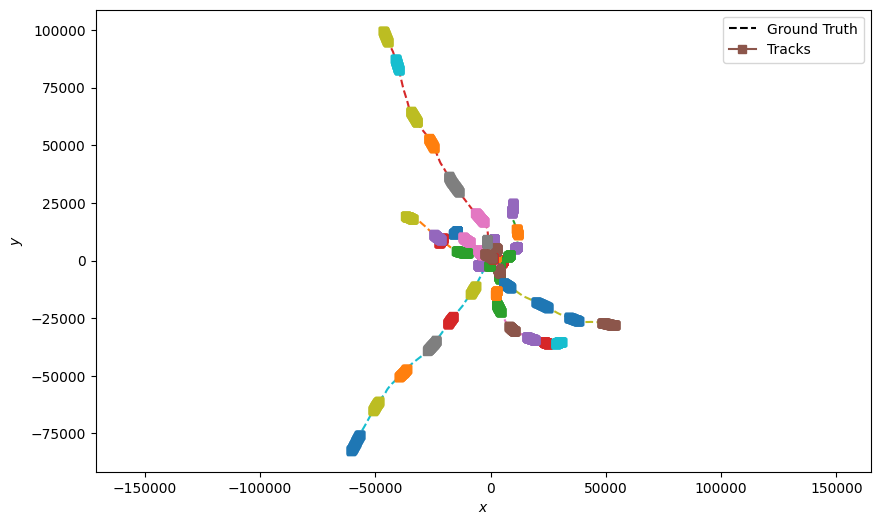•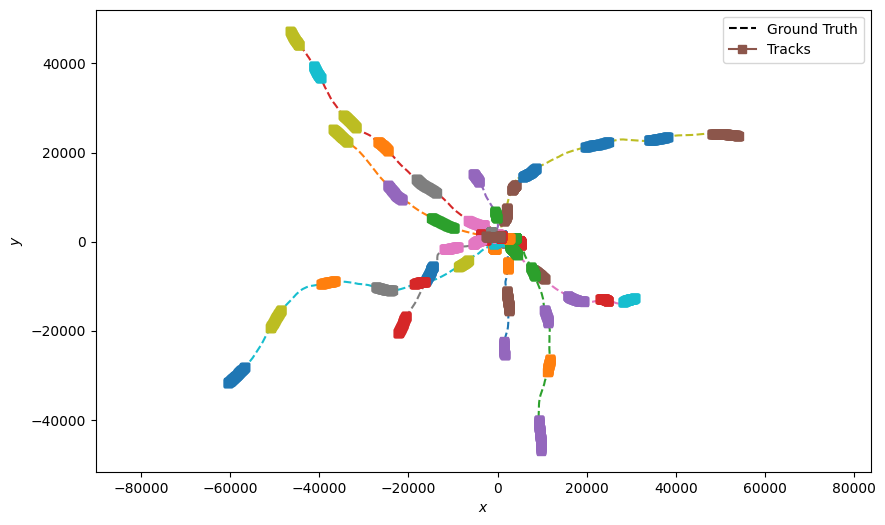•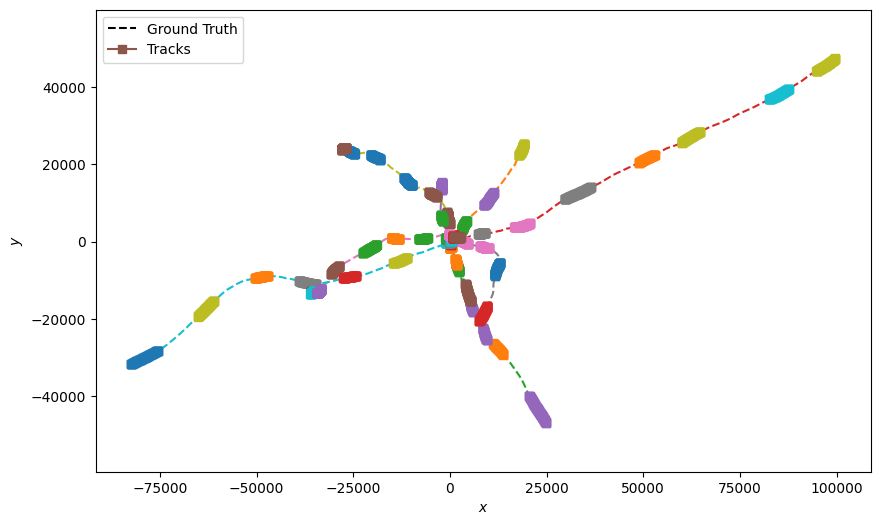# Plot 3D graph if working in 3-dimensional space
if n_spacial_dimensions == 3:
plotter = Plotter(Dimension.THREE)
plotter.plot_ground_truths(truths, [0, 2, 4])
plotter.plot_tracks(all_tracks, [0, 2, 4])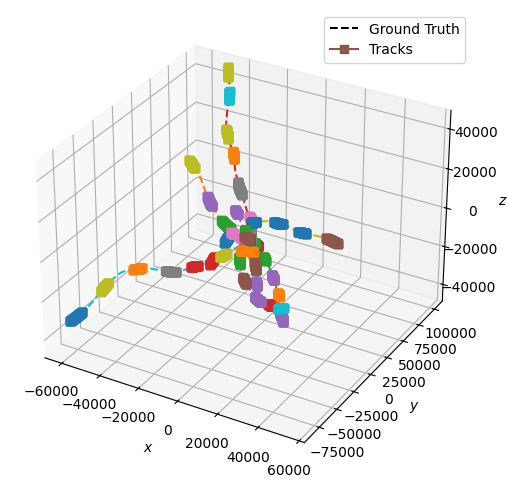### Track Stitcher Class

The cell below contains the track stitcher class. The functions forward_predict and backward_predict perform the forward and backward predictions respectively (as noted above). If using fowards and backwards stitching, predictions from both methods are merged together. They calculate which pairs of tracks could possibly be stitched together. The function stitch uses forward_predict and backward_predict to pair and ‘stitch’ track sections together.

from stonesoup.stitcher import TrackStitcher


### Applying the Track Stitcher

Now that we have a set of tracklets, we can apply the Track Stitching method to stitch tracklets together into tracks. The code in the following cell applies this process using the class TrackStitcher and plots the stitched tracks. TrackStitcher has a property search_window that enables you to reduce compute time by filtering out track segments that are not within a determined time window. When forward stitching, the associator will consider any track that has a start point that falls within the time window $$(t, t + search_window)$$. When backward stitching, the associator will consider tracks that have an endpoint within the time window $$(t - search_window, t)$$.

transition_model = CombinedLinearGaussianTransitionModel([OrnsteinUhlenbeck(0.001, 2e-2)] *
n_spacial_dimensions, seed=12)

predictor = KalmanPredictor(transition_model)
hypothesiser = DistanceHypothesiser(predictor, updater, Mahalanobis(), missed_distance=300)
stitcher = TrackStitcher(forward_hypothesiser=hypothesiser, search_window=timedelta(seconds=500))

stitched_tracks, _ = stitcher.stitch(all_tracks, start_time)

for pair in dim_pairs:
plotter = Plotter()
plotter.plot_ground_truths(truths, list(pair))
plotter.plot_tracks(stitched_tracks, list(pair))

if n_spacial_dimensions == 3:
plotter = Plotter(Dimension.THREE)
plotter.plot_ground_truths(truths, [0, 2, 4])
plotter.plot_tracks(stitched_tracks, [0, 2, 4])

•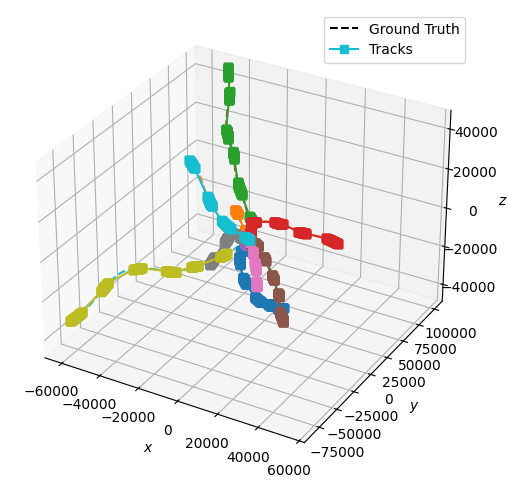•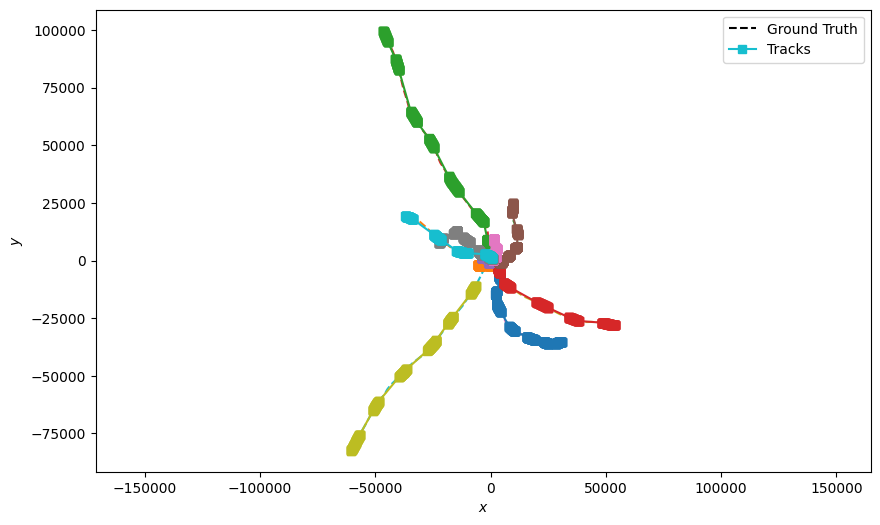•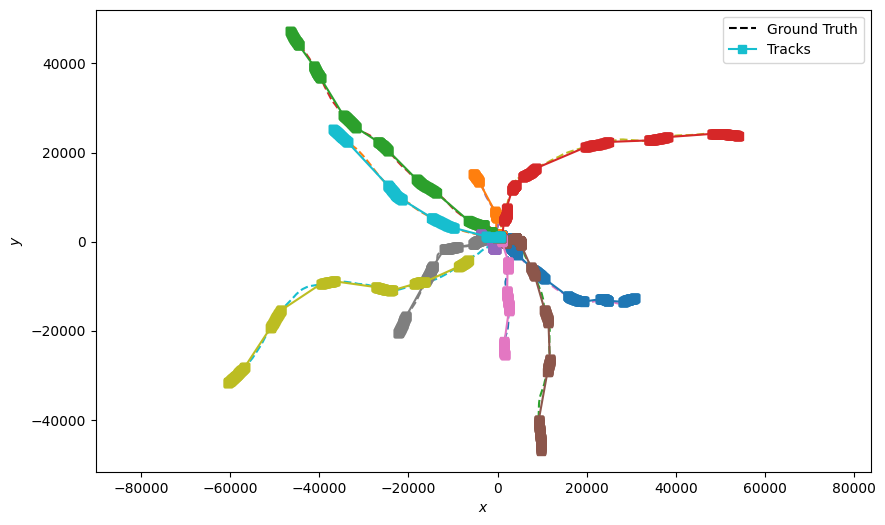•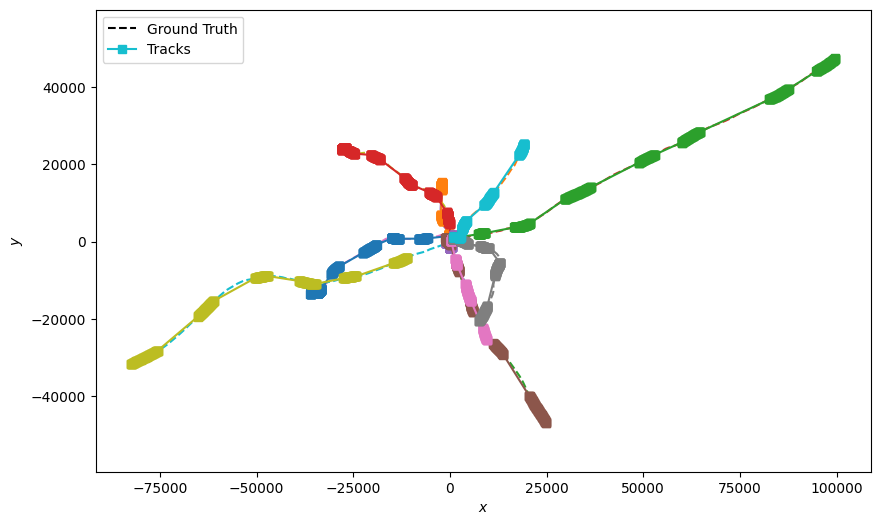## Applying Metrics

Now that we have stitched the tracklets into tracks, we can compare the tracks to the ground truths that were used to generate the tracklets. This can be done by using metrics: find below a range of SIAP (Single Integrated Air Picture) metrics as well as a custom metric specialized for track stitching.

### % of tracklets stitched to the correct previous tracklet

def StitcherCorrectness(stitchedtracks):
stitchedtracks = list(stitchedtracks)
total, count = 0, 0
for track in stitchedtracks:
for j, state in enumerate(track):
if j == len(track) - 1:
continue
id1 = [int(s) for s in state.hypothesis.measurement.groundtruth_path.id.split('::')
if s.isdigit()]
id2 = [int(s) for s in
track[j + 1].hypothesis.measurement.groundtruth_path.id.split('::') if
s.isdigit()]
if id1 != id2:
total += 1
if id1 == id2 and id1 == (id2 - 1):
count += 1
return count / total * 100

print("Tracklets stitched correctly: ", StitcherCorrectness(stitched_tracks), "%")

Tracklets stitched correctly:  89.13043478260869 %


### SIAP Metrics

The following cell calculates and records a range of SIAP (Single Integrated Air Picture) metrics to assess the accuracy of the stitcher. The value of math:association_threshold should be adjusted to represent the acceptable distance for association for the scenario that is being considered. For example, associating with a threshold of 50 metres may be acceptable if tracking a large ship, but not so useful for tracking biological cell movement.

SIAP Ambiguity: Important as a value not equal to 1 suggests that the stitcher is not stitching whole tracks together, or stitching multiple tracks into one.

SIAP Completeness: Not a valuable metric for track stitching evaluation as we are only tracking fractions of the true objects - metric value is scaled by the ratio of truthlets to disjoint sections.

SIAP Rate of Track Number Change: Important metric for assessing track stitching. Any value above zero is showing that tracklets are being incorrectly stitched to tracklets from different truth paths.

from stonesoup.measures import Euclidean
from stonesoup.metricgenerator.tracktotruthmetrics import SIAPMetrics
from stonesoup.dataassociator.tracktotrack import TrackToTruth
from stonesoup.metricgenerator.manager import MultiManager
from stonesoup.metricgenerator.metrictables import SIAPTableGenerator

siap_generator = SIAPMetrics(position_measure=Euclidean((0, 2)),
velocity_measure=Euclidean((1, 3)),
generator_name='SIAPs',
tracks_key='tracks',
truths_key='truths'
)

associator = TrackToTruth(association_threshold=30)

# create metric manager and add tracks and truths data to it
metric_manager = MultiManager([siap_generator],
associator=associator)

# generate metrics and extract SIAP averages to display in SIAP table
metrics = metric_manager.generate_metrics()
siap_metrics = metrics['SIAPs']
siap_averages = {siap_metrics.get(metric) for metric in siap_metrics
if metric.startswith("SIAP") and not metric.endswith(" at times")}

_ = SIAPTableGenerator(siap_averages).compute_metric()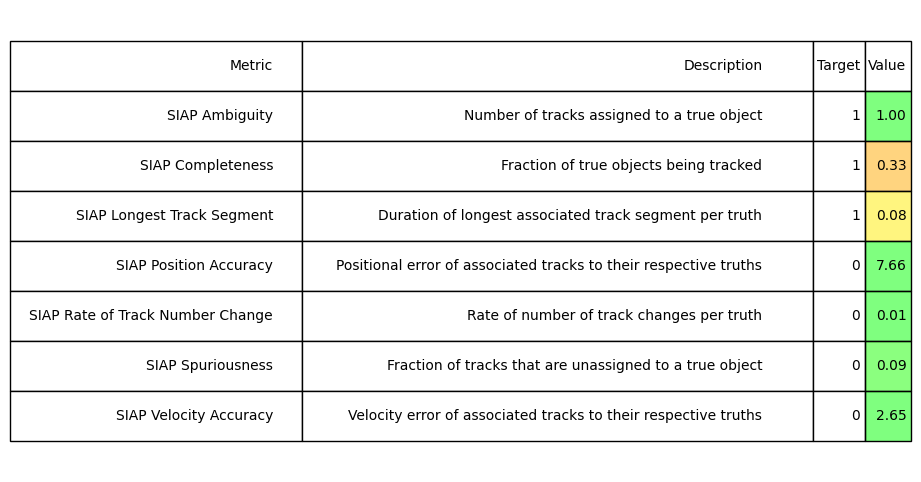Total running time of the script: (0 minutes 56.532 seconds)

Gallery generated by Sphinx-Gallery